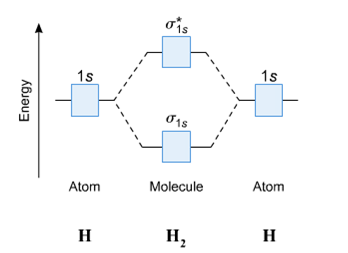# Problem: Construct the molecular orbital diagram for H2 and then identify the bond order. Click within the blue boxes to add electrons.

###### FREE Expert Solution

We will do the following steps

Step 1: Calculate the total number of valence electrons present.

Step 2: Draw the molecular orbital diagram.

Step 3: Calculate the bond order of the molecule/ion. Recall that the formula for bond order is:

84% (394 ratings)###### Problem Details
Construct the molecular orbital diagram for H2 and then identify the bond order. Click within the blue boxes to add electrons.Frequently Asked Questions

What scientific concept do you need to know in order to solve this problem?

Our tutors have indicated that to solve this problem you will need to apply the MO Theory: Bond Order concept. You can view video lessons to learn MO Theory: Bond Order. Or if you need more MO Theory: Bond Order practice, you can also practice MO Theory: Bond Order practice problems.